# Depth First Search (DFS) traversal : Graph

Problem Statement: Given a graph, traverse through all the nodes in the graph using Depth First Search.

```Example:

Input: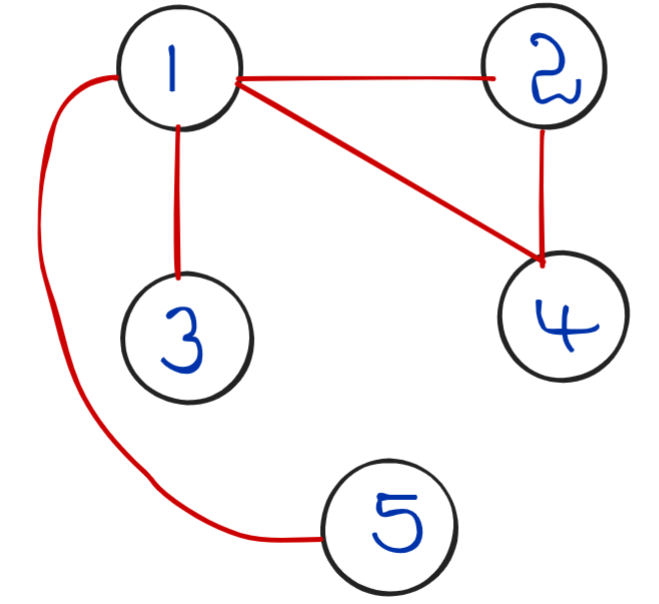Output: 2 4 1 3 5

Explanation: Note: For above I/P we started DFS from Node 2,We can start from any node.
```

# Solution:

DisclaimerDon’t jump directly to the solution, try it out yourself first.

Intuition:

Depth First Traversal is a traversal technique/algorithm, used to traverse through all the nodes in the given graph.

It starts traversal through any one of its neighbour nodes and explores the farthest possible node in each path/branch and then starts Back-tracking.

Backtracking happens when the DFS algorithm reaches a particular node that has no neighbours to visit further, once it reaches that node with no neighbours to be visited it’ll backtrack to its previous node (say N) and from this node N algorithm will start searching for unvisited neighbour nodes and starts visiting them.

Let’s see how it works pictorially;Let’s pick a random node to start the traversal, let it be 2 (You can choose any node).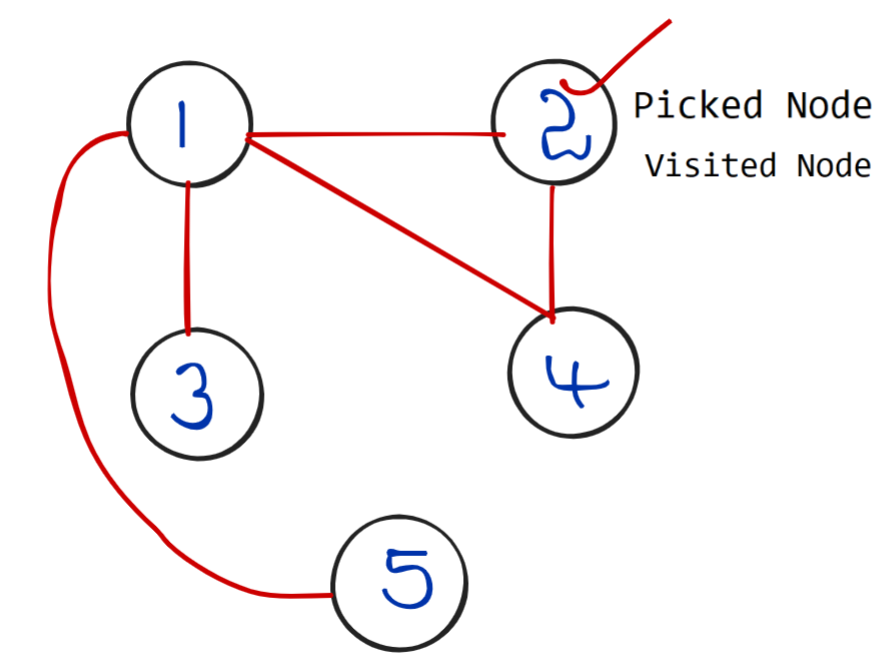• Now, visit any of its neighbour nodes (neighbors of 2 are 1,4) and pick any of its neighbourhood nodes.

Note: Now the Output console has 2 as the answer.

• Now, let’s say the algorithm picks 4.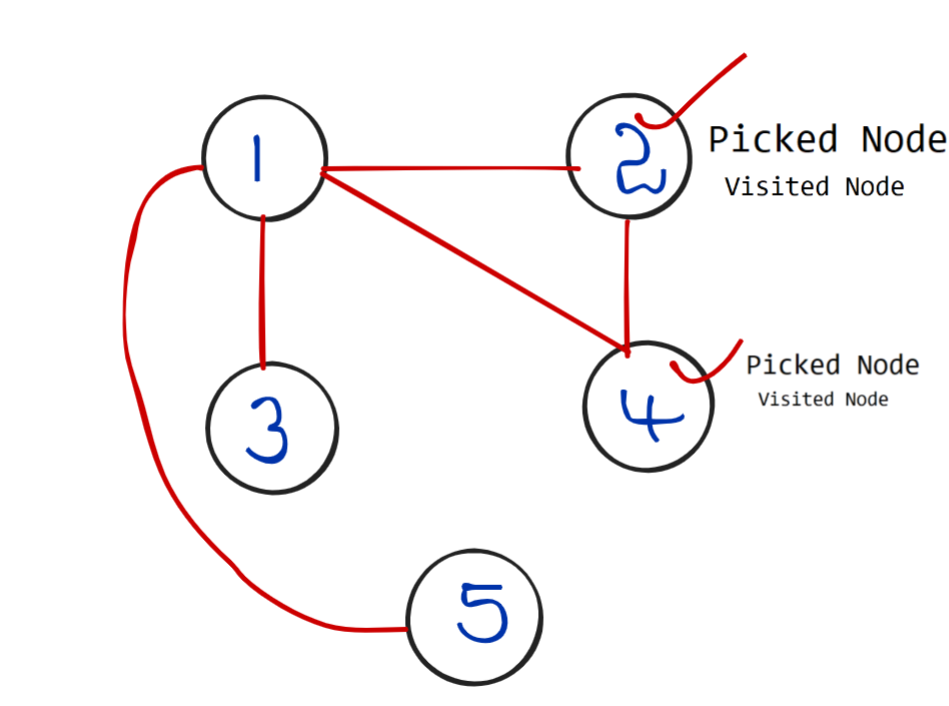• Note : Now the Output console has 2,4 as the answer.
• Now the algorithm checks for the unvisited neighbours of node 4, node 4 has only one unvisited neighbour node i.e 1 , So 1 will be visited.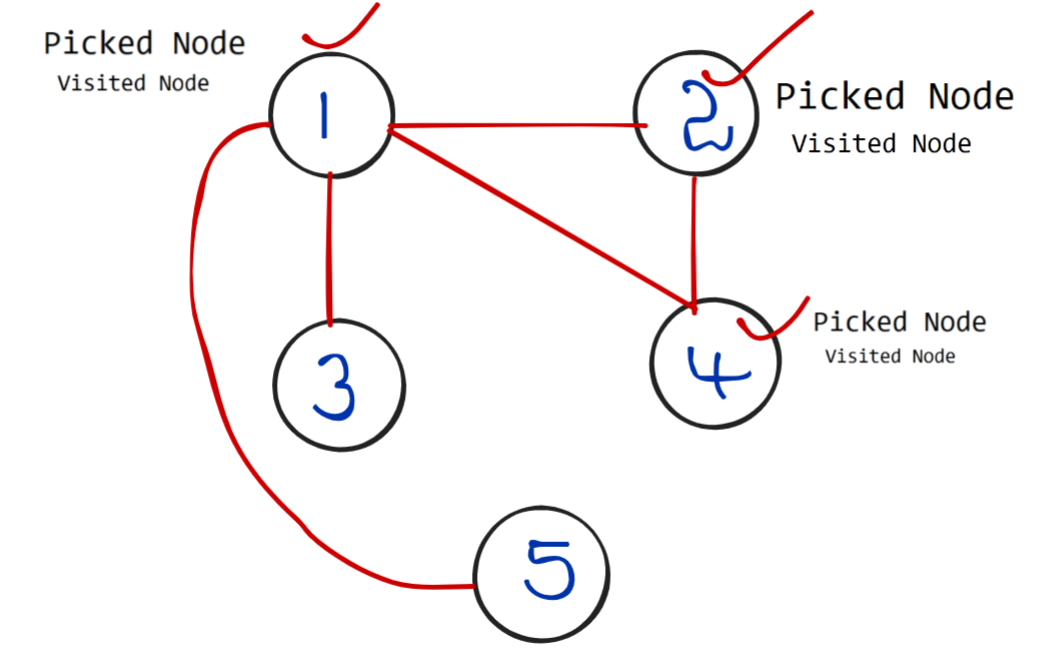Now, neighbours of 1(3,4) that are not visited will be visited, which means repetition of the same process which we did for previous nodes.

• Note : Now the Output console has 2,4,1 as the answer.
• Now let’s assume the algorithm picks 3, so 3 will be visited.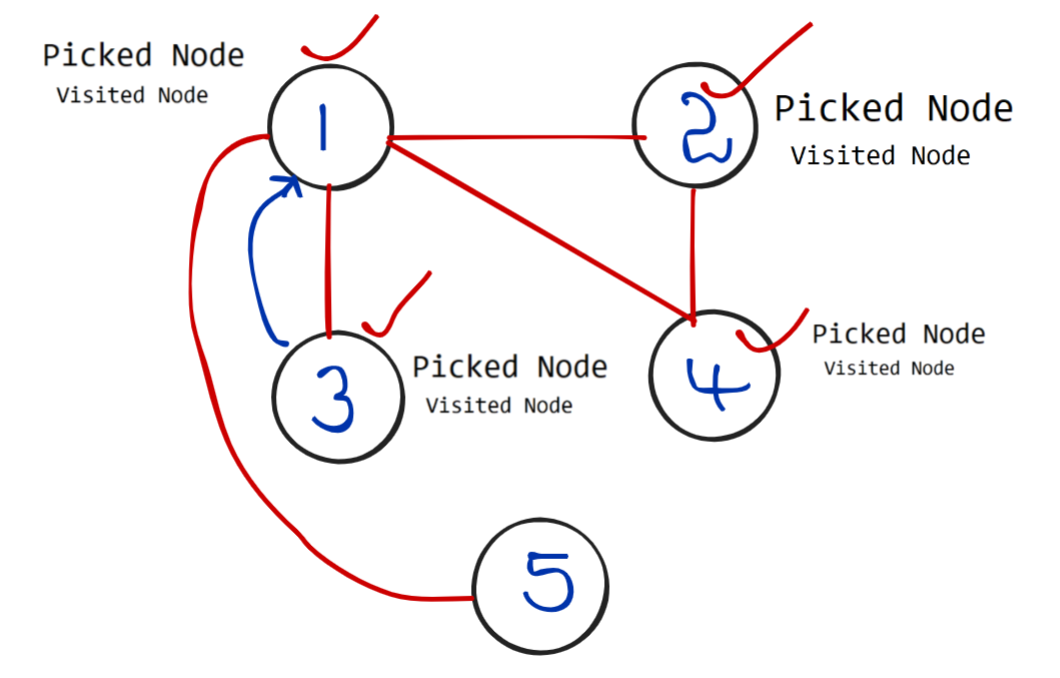Once 3 has been visited, there are no neighbour nodes for 3 which are to be visited so it will backtrack to 1.

• Note : Now the Output console has 2,4,1,3 as the answer.

Now the algorithm is at node 1, when it checks for unvisited neighbours it will only find one node i.e 5, so it will visit 5 and backtrack to 1 as shown below.Note: Now the Output console has 2,4,1,3,5 as the answer, Which is our expected answer!

When it backtracks to 1, now 1 has to backtrack to its previous node and this backtracking will happen till it reaches the start node.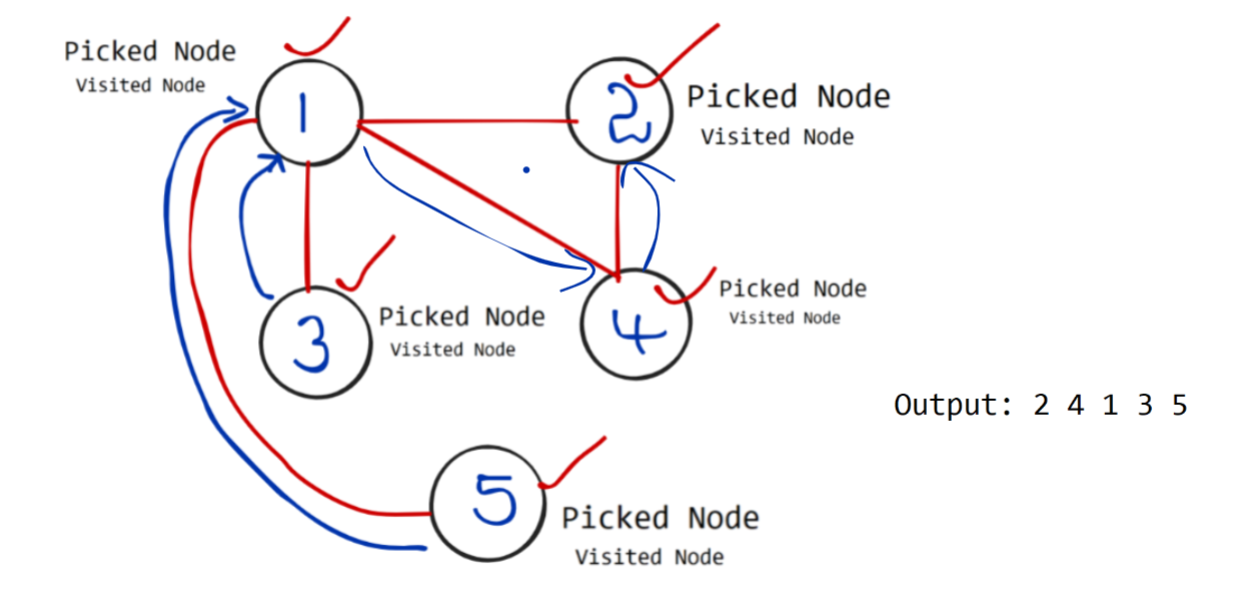Approach:

Once you have gone through the above pictorial representation, you might have got an idea of how to approach this problem, if not let’s see the approach.

1. Start with a random node from graph
2. Make an array to keep track of visited nodes, once visited make that node as visited
3. Print this current node
4. Get  neighbour nodes and perform above steps recursively for each node deeply/depthly if node is unvisited.

Recursion Tree for more understandability :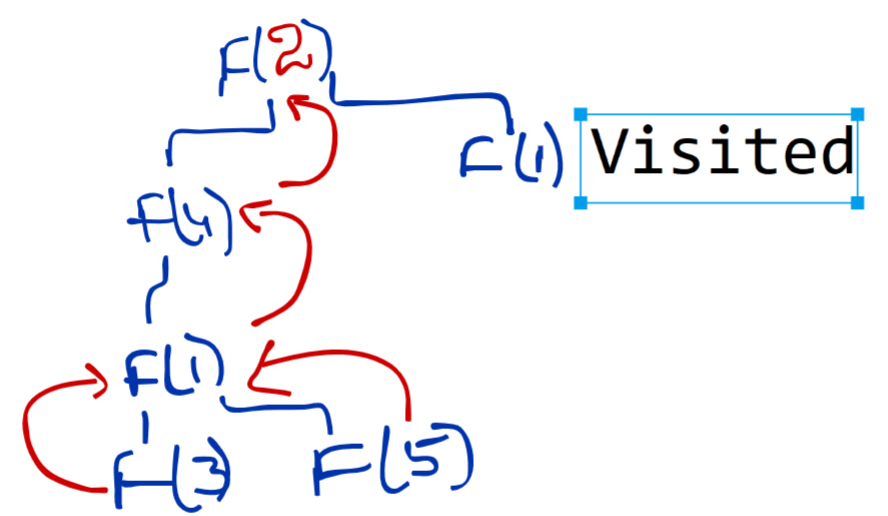Code:

## C++ Code

``````#include <bits/stdc++.h>
using namespace std;
class Solution {
void dfs(int node, vector<int> &vis, vector<int> adj[], vector<int> &storeDfs) {
storeDfs.push_back(node);
vis[node] = 1;
for(auto it : adj[node]) {
if(!vis[it]) {
dfs(it, vis, adj, storeDfs);
}
}
}
public:
vector<int>dfsOfGraph(int V, vector<int> adj[]){
vector<int> storeDfs;
vector<int> vis(V+1, 0);
for(int i = 1;i<=V;i++) {
if(!vis[i]) dfs(i, vis, adj, storeDfs);
}
return storeDfs;
}
};

{
}
int main()
{

Solution obj;
vector<int> df;
for(auto it: df)
cout<<it<<" ";

return 0;
}
``````

Output:

1 2 4 3 5

Time complexity: O(N+E), Where N is the time taken for visiting N nodes and E is for travelling through adjacent nodes.

Space Complexity:O(N+E)+O(N)+O(N) , Space for adjacency list, Array,Auxiliary space.

## Java Code

``````import java.util.*;
class Solution
{
public static void dfs(int node, boolean vis[], ArrayList<ArrayList<Integer>> adj, ArrayList<Integer> storeDfs) {
//marking current node as visited
vis[node] = true;

//getting neighbour nodes
for(Integer it: adj.get(node)) {
if(vis[it] == false) {
dfs(it, vis, adj, storeDfs);
}
}
}
public static ArrayList<Integer> dfsOfGraph(int V, ArrayList<ArrayList<Integer>> adj)
{
ArrayList<Integer> storeDfs = new ArrayList<>();

//boolean array to keep track of visited vertices
boolean vis[] = new boolean[V+1];

//If you are starting from node 2, then i should start from 2.
for(int i = 1;i<=V;i++) {
if(!vis[i]) dfs(i, vis, adj, storeDfs);
}

return storeDfs;
}
static void printAns(ArrayList < Integer > ans) {
for (int i = 0; i < ans.size(); i++) {
System.out.print(ans.get(i) + " ");
}
}
public static void main(String args[]) {

ArrayList < ArrayList < Integer >> adj = new ArrayList < > ();

// adding new arraylists to 'adj' to add neighbour nodes
for (int i = 0; i < 6; i++) {
}

ArrayList < Integer > ans = dfsOfGraph(5, adj);
printAns(ans);
}
}
``````

Output:

1 2 4 3 5

Time complexity: O(N+E), Where N is the time taken for visiting N nodes and E is for travelling through adjacent nodes.

Space Complexity:O(N+E)+O(N)+O(N) , Space for adjacency list, Array,Auxiliary space.

Special thanks to Sai bargav Nellepalli for contributing to this article on takeUforward. If you also wish to share your knowledge with the takeUforward fam, please check out this article